[FrontPage] [TitleIndex] [WordIndex]

# Inner vs. Outer definition on raster data

## 1. Overview

The inner vs. outer problematic is one of the worst cases of the 'off-by-one' paradigm in the OGC world. Currently two different definitions of the origin of an image's pixel are used in the OGC specifications (compare figure 1).

1. The outer definition defines the pixel origin to be on the upper left corner of a pixel (WMS-way).

2. The inner definition defines the pixel origin to be on the center of an image pixel (WCS-way)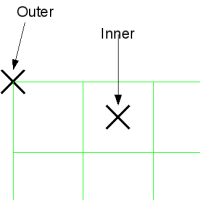While requesting a boundingbox from a 'georeferenced-raster' the result image of this subset may be to large/small if you don't know the position of the origin (center/outer) on the underlying imagery. This document tries to clarify this.

## 2. Raster-API center definition

Like the WCS the deegree3 raster-API is using the CENTER definition internally, was does this mean. Let's assume you have a regular grid of sample points, exemplary displayed in figure 2.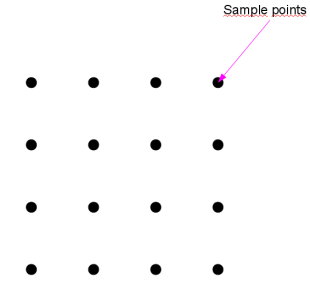These sample points can be anything, height-values, temperatures or anything you can sample. Each sample represents a value for a given domain it is valid for. (see figure 3.)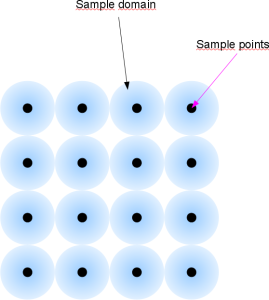By implying a grid with a goereference over these sample points, a georeferenced raster submerges from these mere points, and a Coverage / Raster is extruded (figure 4.)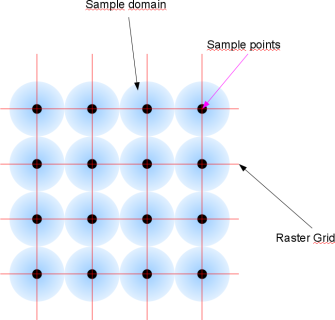Creating an image of these sample points will result in a centered view of the data, each pixel will represent a sample domain with the sample point in the center. (figure 5.) This is the representation of data the coverage api is expecting.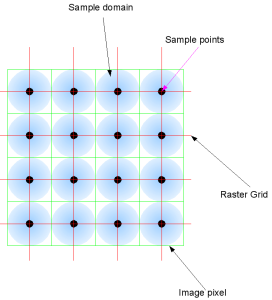The other way around is also true, an image alone is not aware of the geographic location it's values (the pixels) are sampled. You need a Georeference for this. A georeference is not a bounding box, it merely defines the origin of the top left point of the image and the resolution of the axis, the 'geographic size' or 'real world size' each pixel represents (e.g. the sample domain of the sample point). (figure 6).ONLY with this information an image becomes a georeferenced raster from which geo referenced subsets can be extruded.

## 3. Retrieving Subsets from rasters

Only at retrieval of data from a georeferenced raster the center/outer problematic is at hand, figure 7 tries to illuminate the problem.

Let's assume we have a georeferenced raster based on an image, and want to request a subset of the upper left bounding box of this raster, which (by coincidence ;-)) is two times a resolution size. The requested geo envelope in a centered and outer based system is of course the same, but the response image is not. If the origin of the georeference is defined on the center of the upperleft pixel, the requested envelope will result in an image with 9 pixels (two resolutions are span acros three image pixels) in an outer based system the envelopes spans over two pixels.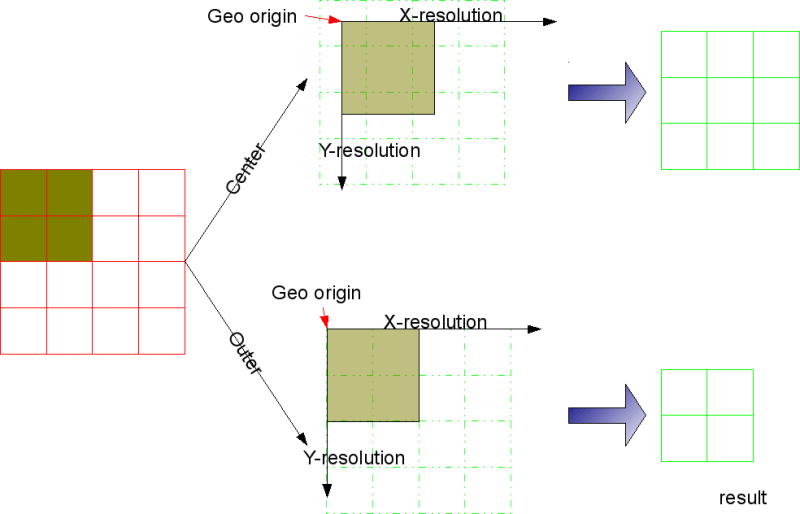Ergo sum, a centered based system can result in an image which is one pixel larger as an outer based image.

2018-04-20 12:05